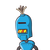# . If 3,8, x and 16 are in proportion the value of x is ( ) A) 12 B) 4 C) 8 D) 6

. If 3,8, x and 16 are in proportion the value of x is ( )
A) 12 B) 4 C) 8 D) 6

1.Step-by-step explanation:

3:8::x:16

multiplying mean digits i.e. 8 and x= 8x

multiplying extreme digits i.e. 16 and 3= 48

8x=48

x=48/8